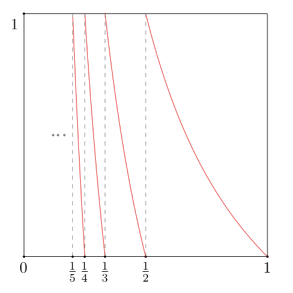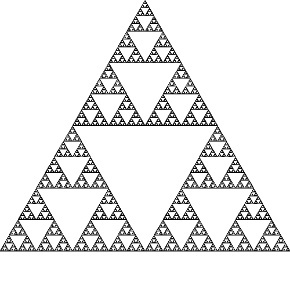Research

One dimensional dynamicsDimension of measures: for a sufficiently nice map of the unit interval $[0,1]$ and an invariant ergodic probability measure $\mu$, the Hausdorff dimension of such measure can be expressed as the quotient between the entropy $h$ and the Lyapunov exponent $\lambda$, that is, $\operatorname{dim}_H \mu = \frac{h}{\lambda}$, when the entropy is finite and the Lyapunov exponent is non-zero. Furthermore, it is known that the local dimension exists almost everywhere and it is also equal to $\frac{h}{\lambda}$. The case when the entropy and the Lyapunov exponent are both zero has been studied by Ledrappier-Misiurewicz and Katok, who constructed examples of such maps and measures for which the local dimension does not exist almost everywhere. I am interested in studying the case when both the entropy and the Lyapunov exponent are infinite.

Dimension of measures with infinite entropy, Nonlinearity 33 (2020) 5355–5382. Journal, Arxiv

In this paper, we prove that for a large class of Expansive-Markov-Renyi (EMR) maps and a Gibbs measure, the lower local dimension is zero almost everywhere (and hence the Hausdorff dimension of the measure is also zero), while the upper local dimension is equal to the convergence exponent of the Markov partition defining the map (and hence, the packing dimension is equal to the convergence exponent). We also prove that the symbolic dimension exists and is also equal to the convergence exponent of the map. The class of maps considered includes the Gauss map, generalized Lüroth maps as well as a large class of maps modelled by countable Markov shifts.

The results from the paper above show that it is possible to develop a theory in this setting.

Limit laws of sequential and random dynamical systems:

The classical limit laws apply for sequences of random variables with some degree of stationarity. For instance, a Law of Large Numbers and a Central Limit Theorem hold for a class of martingales and a class of ergodic stationary processes. We can extend these notions by considering processes arising from sequential and random compositions of different dynamical systems acting in the same space. The resulting systems are non-stationary. While several laws have already been proved, I am interested in Large Deviations and Central Limit Theorems for such systems.

Large deviations and central limit theorems for sequential and random systems of intermittent maps, joint with Matthew Nicol and Andrew Torok (2020), Ergodic Theory and Dynamical Systems, 1-28. doi:10.1017/etds.2020.90 Link in journal (open read access) https://arxiv.org/abs/1909.07435

We obtain large deviations estimates for both sequential and random compositions of intermittent maps. We also address the question of whether or not centering is necessary for the quenched central limit theorems (CLT) obtained by Nicol, Török and Vaienti for random dynamical systems comprised of intermittent maps. Using recent work of Abdelkader and Aimino, Hella and Stenlund we extend the results of Nicol, Török and Vaienti on quenched central limit theorems (CLT) for centered observables over random compositions of intermittent maps: first by enlarging the parameter range over which the quenched CLT holds; and second by showing that the variance in the quenched CLT is almost surely constant (and the same as the variance of the annealed CLT) and that centering is needed to obtain this quenched CLT.

Two dimensional dynamicsSelf-affine measures for self-affine iterated functions systems (IFS), the Hausdorff dimension of the underlying self-affine set can be expressed in terms of the subadditive pressure (associated to the Falconer potential). The measures of equilibrium for such pressure are known as Käenmäki measures. I am interested in studying the statistical properties of such measures.

PhD Thesis

Here is my PhD thesis. It basically contains the work described above in more details, as well as some other results that have not been published yet.

These notes by Vitaly Bergelson give insightful historical remarks on the ergodic theorem.

My research: here I explain my research, the area of investigation and the kind of questions we try to answer. This is written in a format accessible for non-mathematicians.

Chaos and order: here. This is the sketch of the talk that I have in the 3 Minute Thesis competition, which was chosen for the final stage. The video of the talk can be found here.

Juegos que son justos pero no deberían serlo a talk about infinite probability that I gave for the students seminar of my former university, UC Chile. The video of the talk can be found here. In spanish.

Quantum astronomy: matemáticas y la dimensión fractal podcast hosted by astronomers Simón Angel and Luis Rodríguez, where I talked about dynamical systems, ergodic theory and fractal geometry in an accessible way for any stem undergrad. Link of the talk (in spotify) here and the video here.

My Master’s thesis: here. I give a general introduction to ergodic theory with emphasis in Thermodynamic Formalism. In the last chapter, I prove that the dimension of the Borel-Bernstein depends analytically on the parameter.

Slides of my thesis presentation (in Spanish): here. These are the slides I used when presenting my Master’s thesis. They contain a summarized version of the results of my thesis, as well as some ideas of the proof.

Infinite entropy and zero dimensional measures (poster): here. This is a poster I presented at several conferences.

Sizing small sets: here. These are the slides of a talk that I gave in the Pure Postgraduate Seminar. Here I introduce the notion of Hausdorff dimension and how can it be used to compare sizes of sets of measure zero. I present some number theoretical results concerning dimensions of certain sets, as well as sketch of the connection to dynamical systems and ergodic theory.

My talk is full of eels: here These are the slides of a talk that I gave in the Pure Postgraduate Seminar. Here I talked about the Buffon’s needle problem and how the linearity of expectation yields unexpected consequences.